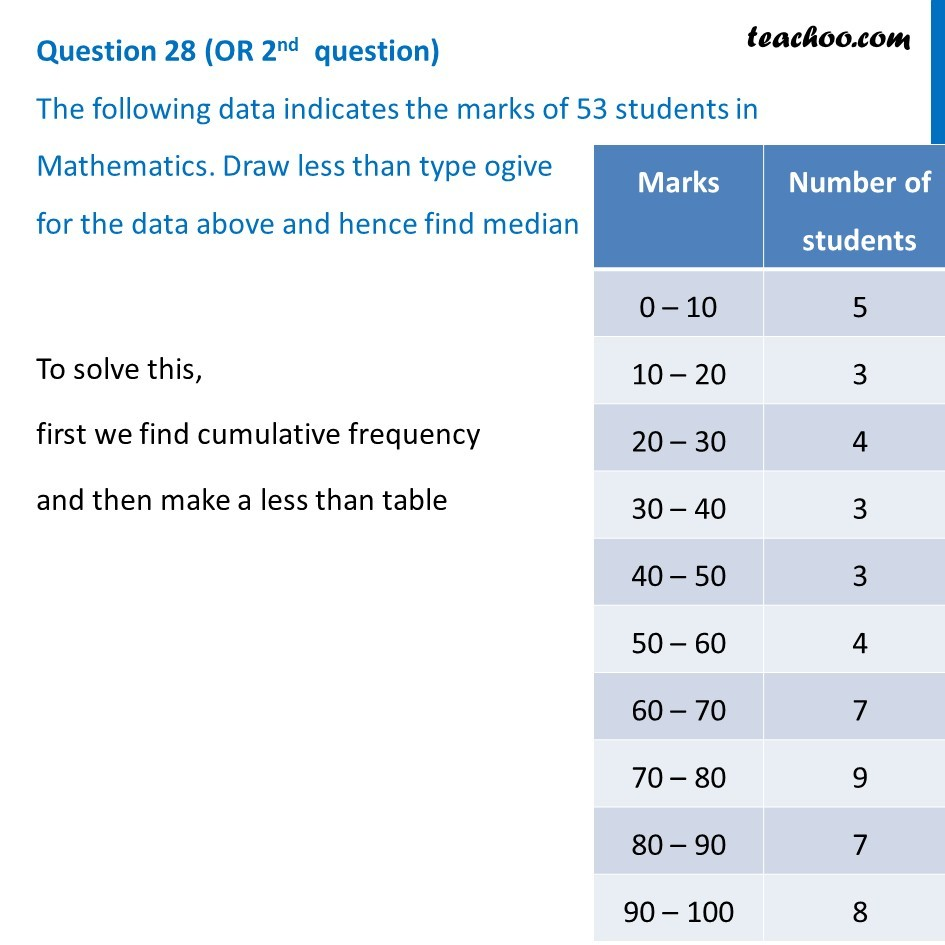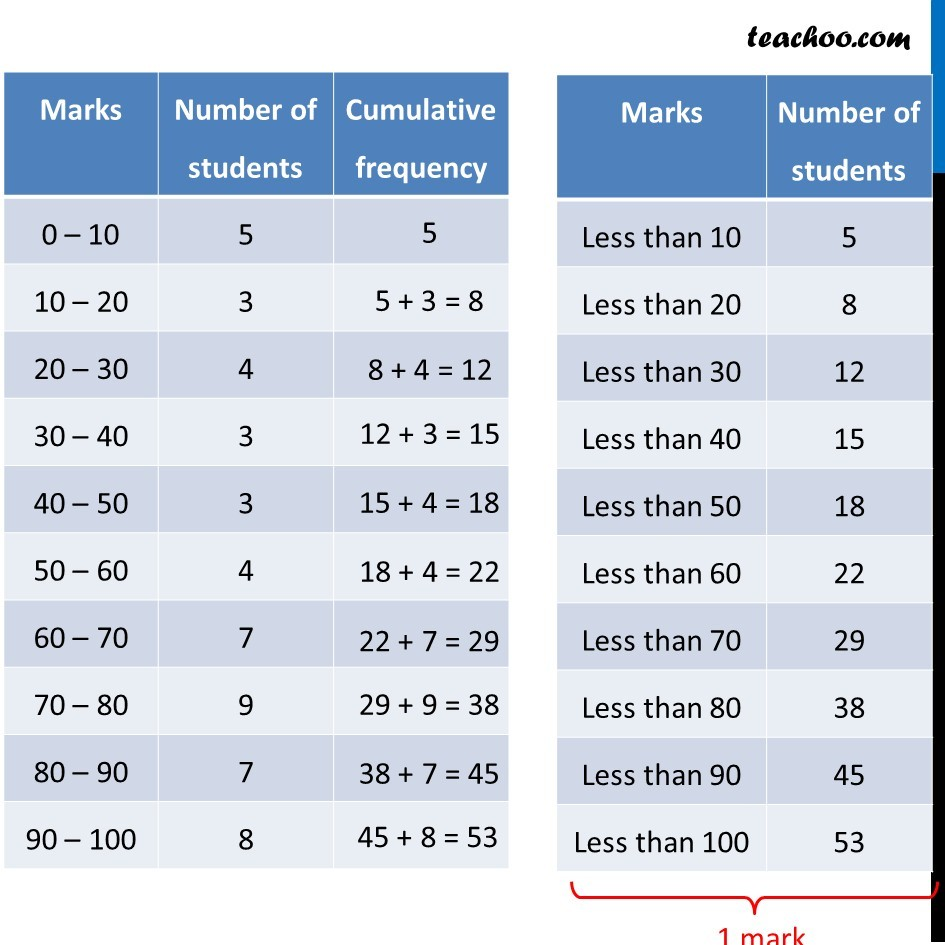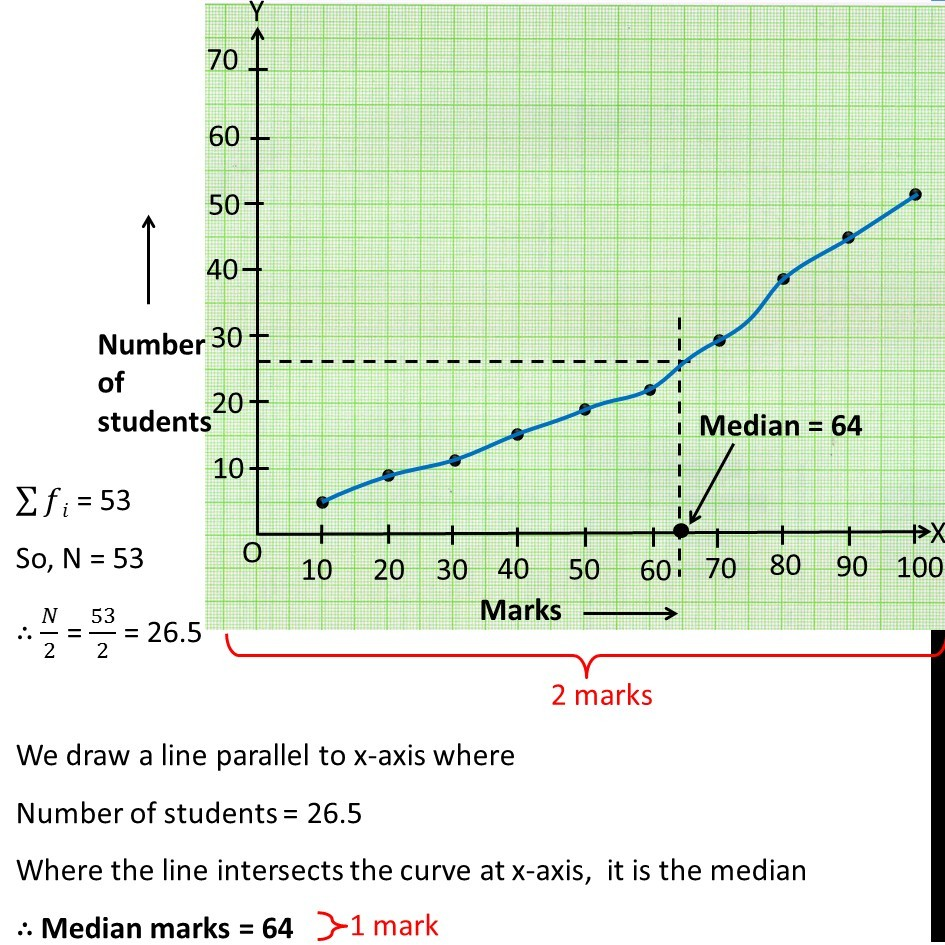CBSE Class 10 Sample Paper for 2019 Boards

Class 10
Solutions of Sample Papers for Class 10 Boards

Question 28 (OR 2 nd   question)

The following data indicates the marks of 53 students in Mathematics. Draw less than type ogive for the data above and hence find median

 Marks Number of students 0 – 10 5 10 – 20 3 20 – 30 4 30 – 40 3 40 – 50 3 50 – 60 4 60 – 70 7 70 – 80 9 80 – 90 7 90 – 100 8Learn in your speed, with individual attention - Teachoo Maths 1-on-1 Class

### Transcript

Question 28 (OR 2nd question) The following data indicates the marks of 53 students in Mathematics. Draw less than type ogive for the data above and hence find median To solve this, first we find cumulative frequency and then make a less than table ∑▒𝑓𝑖 = 53 So, N = 53 ∴ 𝑁/2 = 53/2 = 26.5 We draw a line parallel to x-axis where Number of students = 26.5 Where the line intersects the curve at x-axis, it is the median ∴ Median marks = 64